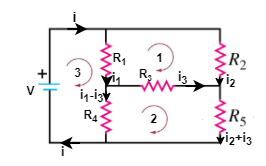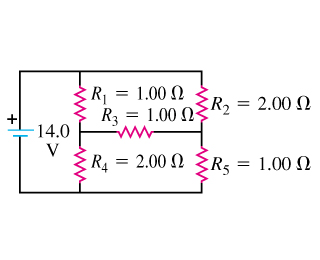# Problem: ​​A) Find the current through the battery in the circuit shown in the figure (Figure 1) .B) Find the current through the resistor R1 in the circuit.C) Find the current through the resistor R2 in the circuit.D) Find the current through the resistor R3 in the circuit.E) Find the current through the resistor R4 in the circuit.F) Find the current through the resistor R5 in the circuit.

###### FREE Expert Solution

Kirchhoff's voltage law:

$\overline{){\mathbf{\Sigma }}{\mathbf{V}}{\mathbf{=}}{\mathbf{0}}}$

From Ohm's law:

$\overline{){\mathbf{V}}{\mathbf{=}}{\mathbf{i}}{\mathbf{R}}}$

Let's label the diagram as follows:97% (92 ratings)###### Problem Details

A) Find the current through the battery in the circuit shown in the figure (Figure 1) .

B) Find the current through the resistor R1 in the circuit.

C) Find the current through the resistor R2 in the circuit.

D) Find the current through the resistor R3 in the circuit.

E) Find the current through the resistor R4 in the circuit.

F) Find the current through the resistor R5 in the circuit.Frequently Asked Questions

What scientific concept do you need to know in order to solve this problem?

Our tutors have indicated that to solve this problem you will need to apply the Kirchhoff's Loop Rule concept. You can view video lessons to learn Kirchhoff's Loop Rule. Or if you need more Kirchhoff's Loop Rule practice, you can also practice Kirchhoff's Loop Rule practice problems.